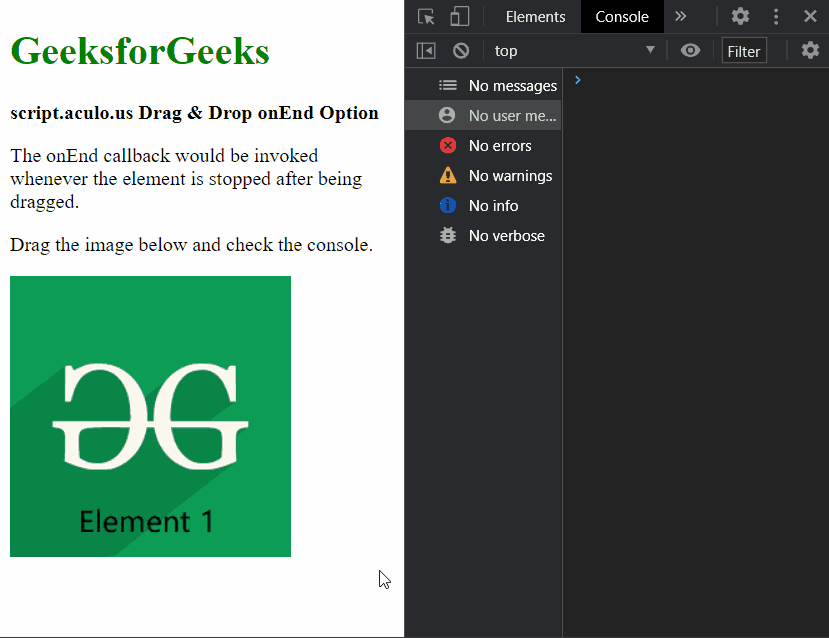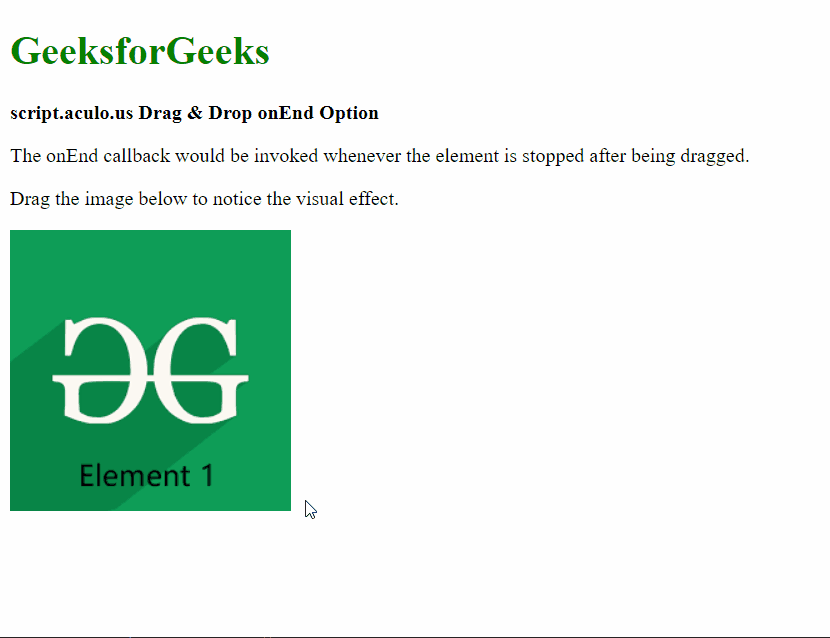Related Articles
script.aculo.us Drag & Drop onEnd Option
• Last Updated : 25 Dec, 2020

The script.aculo.us library is a cross-browser library that aims at improving the user interface of a website. The Drag & Drop module can be used to make any element drag-gable and also enables them to be dropped in a drop zone.

The onEnd option is used to specify a callback function that would be invoked whenever the dragging of the element ends. The element which is dragged would be passed as the parameter to the function.

Include Scripts:

Step 1: Before getting started, You will require to download the script files which are included in the <head> tag of our HTML page.  You can download it from http://script.aculo.us/downloads

Step 2: Unzip the files and put the required files (mainly prototype.js and scriptaculous.js) in the current root directory of your folder.

Step 3: Place any image of your choice in the current root directory of your folder, like in the following example elem1.png is used.

Syntax:

`{ onEnd: function }`

Parameters: This option has a single value as mentioned above and described below:

• function: This is a callback function that would be invoked whenever the dragging of an element ends.

The below examples illustrates the use of this option.

Example 1:

## HTML

 ```<``html``>``<``head``>``  ``<``script` `type``=``"text/javascript"` `    ``src``=``"prototype.js"``>``  ````  ``<``script` `type``=``"text/javascript"` `    ``src``=``"scriptaculous.js"``>``  ```` ` `  ``<``script` `type``=``"text/javascript"``>``    ``window.onload = function () {`` ` `      ``// Define a function to be used``      ``// when the element stops after``      ``// being dragged``      ``new Draggable('elem1', {``        ``onEnd: (elem) => {``          ``console.log(``            ``"The dragging of the element has stopped"``          ``);``          ``console.log(elem);``        ``}``      ``});``    ``};``  `````` ` `<``body``>``  ``<``div``>``    ``<``h1` `style``=``"color: green"``>``      ``GeeksforGeeks``    ````  ````  ``<``strong``>``    ``script.aculo.us Drag &``    ``Drop onEnd Option``  ````   ` `  ``<``p``>``    ``The onEnd callback would be``    ``invoked whenever the element ``    ``is stopped after being dragged.``  ````   ` `  ``<``p``>``    ``Drag the image below and``    ``check the console.``  ```` ` `  ``<``img` `id``=``"elem1"` `src``=``"elem1.png"``>`````

Output:Example 2:

## HTML

 ```<``html``>`` ` `<``head``>``  ``<``script` `type``=``"text/javascript"` `    ``src``=``"prototype.js"``>``  ````  ``<``script` `type``=``"text/javascript"` `    ``src``=``"scriptaculous.js"``>``  ```` ` `  ``<``script` `type``=``"text/javascript"``>``    ``window.onload = function () {``      ``new Draggable('elem1', {`` ` `        ``// Define a function to be used``        ``// when the element stops ``        ``// to be dragged``        ``onEnd: () => {``          ``new Effect.Squish('elem1',``          ``);``        ``}``      ``});``    ``};``  ``````<``body``>``  ``<``div``>``    ``<``h1` `style``=``"color: green"``>``      ``GeeksforGeeks``    ````  ````  ``<``strong``>``    ``script.aculo.us Drag &``    ``Drop onEnd Option``  ````   ` `  ``<``p``>``    ``The onEnd callback would be ``    ``invoked whenever the element is``    ``stopped after being dragged.``  ```` ` `  ``<``p``>``    ``Drag the image below to notice``    ``the visual effect.``  ```` ` `  ``<``img` `id``=``"elem1"` `src``=``"elem1.png"``>```` ` ``

Output:Reference: http://script.aculo.us/My Personal Notes arrow_drop_up